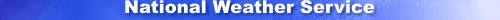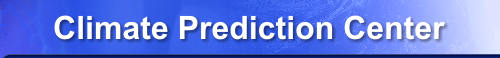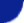Site Map News Organization SearchHOME > Monitoring_and_Data > Oceanic and Atmospheric Data > Reanalysis: Atmospheric Data > wgrib2-rpn

Introduction

The -rpn option runs a reverse polish notation (RPN) calculator. Having a built-in calculator is quite handy. We use it to convert units (ex. geopotential to geopotential meters, accumulations to rates), compute simple quantities (net flux from downward and upward fluxes), and even compute the plant hardiness index from the 2 m temperatures. The goal of the calculator is to reduce the need to write simple grib programs that do simple calculations.

The "hardware" of the rpn calculator consists of 20 registers and a stack (10 entries deep). (Wgrib2 prior to 2.0.6 has 10 registers.) Stack entries and registers are arrays rather floating point numbers on your store-bought calculator.

The conceptual model of the >-rpn calculator is the grid values array is the top of the stack. The calculator has a statck that is 10 entries deep and 20 registers. When ever you enter rpn mode, the stack is cleared except for the top of the stack. The registers are only clears when you "turn on" the calculator; that is start the wgrib2 utility or the first call to wgrib2 if you are using calling wgrib2 through wgrib2api or pywgrib2.

To save the calculations, you can save them in a register or write them out by -grib_out, -bin, -ieee, -text, etc.

Callable wgrib2, wgrib2api

Callable Wgrib2 and wgrib2api use the -rpn registers to transfer gridded data between the calling program and the wgrib2 subroutine. A Fortran or C program can read and write the wgrib2's RPN registers. For example, if a program wants to write a grib2 file, it would first place the grid values into a register. The calling program would then call the wgrib2 subroutine with instructions to read a template, replace the grid values with the register values, and then write a grib message.

Implementation Details

The size of a registers may differ as the size of grids can also vary in a grib file. However the size of the register has to match the size of the stack entry in order "recall" the register.

Wgrib2 always reads a grib message before processing it using commmands like -rpn. This sets the size of the data array. Thus the size of the stack entries is always the size of the grib message that is being processed.

The conceptual moddel is the data array (grid values) is the top of the stack. The implmentation is that the data array is copied to the top of the stack when -rpn is run, and the top of the stack is copied to the data array when -rpn is finished. An error message will occur if the top of the stack is empty when -rpn finishes.

Limitations of -rpn

The -rpn option was designed for simple calculations. For more complicated calculations, you should use a real programming language. You can do the calculations in another step and then import the results using one of the various -import_* options. You can use wgrib2api (Fortran and C) to read the data into a Fortran or C program, do the calculation and then write the data out using wgrib2. Finally you can use python and one of the pywgrib2 packages to do the calculation using Python, numpy and pywgrib2.

Uses

• change of units when importing data (gribifying data)
• computations: ex, U,V -> wind speed, wind direction, potential temperature
• merging data
• changing units before writing text/ieee files
• removing extreme data values
• finding min and max values
• finding the globally averaged precipitation
• comparing fields

Usage

-rpn  "A:B:C:..."
A,B,C,.. = number, rpn function, or rpn operator

Operators and Functions:

Pop X, Push Fn(X)
• abs: absolute value
• acos: arc cos, [0, pi] radians
• alt_x_scan: changes alternate x scanning to regular x scanning and vice versa
• asin: arc sin, result is [-pi/2, pi/2] radians
• atan: arc tan, result is [-pi/2, pi/2] radians, see atan2
• abs: absolute value
• ceil: smallest integer >= X
• cos: cosine, argument in radians
• exp: e^X
• floor: largest integer <= X
• ln: natural logorithm
• raw2: convert from input scan order to output scan order
• sin: sine, argument in radians
• smth9g: smth9 for global fields
• smth9r: snth9 for regional fields
• sq: X*X
• sqrt: square root
• tan: tangent, argument in radians
• xave: for nx-ny grids, averages in the x direction (normally zonal mean)
• xdev: for nx-ny grids, remove x average (normally deviation from zonal mean)
• yrev: for nx-ny grids, changes we:sn to we:ns and vice versa
• 1/x: 1/X
• 2raw: convert from output scan order to input scan order
Pop Y, Pop X, push Fn(X,Y)
• +: push X+Y
• -: push X-Y
• *: push X*Y
• /: push X/Y
• <: push X < Y (1/0 if true/false)
• <=: push X <= Y (1/0 if true/false)
• ==: push X == Y (1/0 if true/false)
• !=: push X != Y (1/0 if true/false)
• >=: push X >= Y (1/0 if true/false)
• >: push X > Y (1/0 if true/false)
• atan2: push arctan(X/Y), result is [-pi, pi] radians, see atan
• pow: push X**Y (X^Y)
• mask: if (Y != 0) push(X) else push(UNDEFINED)
• max: push max(X,Y), for logical values, max is the same as OR
• merge: if (Y != UNDEFINED) push(Y) else push(X)
• min: push min(X,Y), for logical values, min is the same as AND
Note: an operation involving an UNDEFINED is UNDEFINED
Stack Operators:
• clr, clear the stack (stack is emptied)
• dup, duplicate the top of the stack
• pop, remove the top of the stack
• exc/swap, exchange the top 2 stack entries
Register Operators: (note: CW2 v2.0.6+ uses registers 7,8,9 prior versions 0,1,2)
• clr_I, clear register I, I=0,1..,9 (19 for v2.0.6+)
• rcl_I, push register I on top of stack, I=0,1..,9 (19 for v2.0.6+)
• sto_I, save top of stack in register I, I=0,1..,9 (19 for v2.0.6+)
• rcl_lat, push latitudes onto the top of the stack (degrees)
• rcl_lon, push longitudes onto the top of the stack (degrees)
• sto_lon, save top of stack as longitudes (degrees) (wgrib2 v3.0.0+)
• sto_lat, save top of stack as latitudes (degrees) (wgrib2 v3.0.0+)
• note: latitudes and longitudes are double precision values, the stack is single precision
Variables and Constants: put on the top of the stack
• number number = floating point or integer number like 0, 10.1, -1.23e-4
• days_in_ref_month number of days in the month for the reference date (conversion between monthly acc. and rates)
• days_in_verf_month number of days in the month for the verification time (conversion betwee monthly acc. and rates)
• pi 3.1415....
• rand random number uniformly distributed between 0 and 1, each grid point has a different random number
Printing Operators:
• print_corr, write cosine weighted spatial correlation, data[TOP] data[TOP-1]
• print_max, print_min, data[TOP]
• print_rms, write cosine weighted RMS, data[TOP]-data[TOP-1]
• print_diff, write cosine weighted difference, data[TOP-1]-data[TOP]
• print_ave, write cosine weighted average, grid_ave(data[TOP]*cos(grid))/grid_ave(cos(grid))
• print_wt_ave, write weighted average grid_ave, data[TOP] is the weighting print grid_ave(data[TOP]*data[TOP-1])/grid_ave(data[TOP])

Example 1

The standard units of grib temperature is K but you want the text output in Celcius.

\$ wgrib2 a.grb -match ":TMP:850 mb:" -rpn "273.15:-" -text C.dat

Fahrenheit is easy too (F = (K-273.15)*9/5+32).

\$ wgrib2 a.grb -match ":TMP:850 mb:" -rpn "273.15:-:9:*:5:/:32:+" -text F.dat

Example 2

Suppose you want to limit the relative humidity values to 100. This example only affect the RH fields. All submessages will be converted into messages.

\$ wgrib2 a.grb -if ":RH:" -rpn "100:min" -fi -grib_out out.grb -not_if ":RH:" -grib out.grb

Example 3

Suppose that you wanted the 500 to 1000 mb thickness, and the file only contained one field of Z1000 and one field of Z500.

\$ wgrib2 IN.grb -match ":HGT:" -match ":(500|1000) mb:" \
-if ":500 mb:" -rpn sto_1 -fi \
-if ":1000 mb:" -rpn sto_2 -fi \
-if_reg "1:2" \
-rpn "rcl_1:rcl_2:-:clr_1:clr_2" \
-set_var THICK -set_lev "500-1000 mb" \
-set_grib_type c3 -grib_out OUT.grb

line 1: only process the HGT at 500 and 1000 mb which save processing time
line 2: store HGT at 500mb in register 1
line 3: store HGT at 1000mb in register 2
line 4: if (register 1 and register 2 have values then
line 5: calculate the thickness: reg_1 - reg_2
line 6: set variable type to THICK, and level to "500-1000 mb"
line 7: write out the WIND data to a grib file with complex compression

Note: this is a very simple script and that doesn't check the matching
date code, grid type, etc.

Example 4

Write out the 500 mb wind speed.

\$ wgrib2 IN.grb -match ":[UV]grd:500 mb:" \
-if ":UGRD:" -rpn "sto_1" -fi \
-if ":VGRD:" -rpn "sto_2" -fi \
-if_reg 1:2 \
-rpn "rcl_1:sq:rcl_2:sq:+:sqrt:clr_1:clr_2" \
-set_var WIND \
-grib_out out.grb

line 1: only process the U and V at 500 mb
line 2: store U 500mb in register 1
line 3: store V 500mb in register 2
line 4: if (register 1 and register 2 have values then
line 5: calculate the wind speed: sqrt(reg_1**2 + reg_2**2)
line 6: set variable type to WIND (wind speed)
line 7: write out the WIND data to a grib file

Note: this is a very simple script and that doesn't check the matching
date code, grid type, etc.

Note: there are options to calculate wind speed and wind direction

Example 5

Suppose someone made a mistake and the latent heat flux (LHTFL) had the wrong sign. RPN to the rescue.

\$ wgrib2 IN.grb -match ":LHTFL:" -rpn "-1:*" -grib_out new_lhtfl.grb
The file, new_lhtfl, only contained the LHTFL fields. You duplicate the file with the fixed LHTFL fields by
\$ wgrib2 IN.grb -if ":LHTFL:" -rpn "-1:*" -fi -grib_out new.grb
It would be faster if you only compressed the LHTFL fields. (-grib uses the original compressed data and -grib_out uses the "data" register.)
\$ wgrib2 IN.grb -set_grib_type jpeg \
-not_if ":LHTFL:" -grib new.grb -if ":LHTFL:" -grib_out new.grb
If both the latent and sensible heat fluxes needed a sign reversal, you could do,
\$ wgrib2 IN.grb -if ":(LHTFL|SHTFL):" -rpn "-1:*" -fi -grib_out new.grb

Example 6

If you want to set certain values to undefined, you define a mask and then apply the mask. In this example, values below 20 are set to undefined.
\$ wgrib2 a.grb -rpn "dup:20:>=:mask" -grib_out -set_grib_type c3 new.grb

The RPN calculator is used:
dup       the data is duplicated
20        20 is pushed on the stack
>=        test data >= 20, top of stack is 1/0 depending on test >= 20

-set_grib_type c3    sets the grib compression to complex3
-grib_out new.grb    writes a grib message using the decoded data
Don't forget to enclose the argument to rpn in quotes because the shell can do unexpect things. Printing operators
print_corr   write cosine weighted spatial correlation R(TOP-1), R(TOP)
print_max    write max(R(TOP)) to stdout
print_min    write min(R(TOP)) to stdout
print_rms    write cosine weighted RMS(R(TOP-1)-R(TOP))

Example 7: Merging

Suppose that we have a nested model, we have a low resolution TMP2m from the the outer model and a high-resolution TMP2m from the nested model. Now we want a field that uses the TMP2m in the nested-model domain and the TMP2m from the outer model elsewhere. To do this, you need to convert both fields to a common grid. Then you use "-rpn merge". Make sure that both domains are contained in the common grid as this is a requirement of the interpolation library.

wgrib2 OUTER.T2m -new_grid_winds earth -new_grid A B C A1.grb
wgrib2 NESTED.T2m -new_grid_winds earth -new_grid A B C A2.grb
wgrib2 A2.grb -rpn sto_1 -import_grib A1.GRB -rpn "rcl_1:merge" \
-grib_out MERGED.T2m

The file mask.grb contains the values 0 for water, 1 for land and 2 for sea ice. I wanted a small file with 0 for water+sea-ice and 1 for land.
wgrib2 mask.grb -rpn "1:==" -set_scaling 0 1 -set_grib_type c1 -grib_out land.grb

-rpn "1:=="        if grid value is 1, the new value is 1 else 0
-set_scaling 0 1   1 bit for storing the grib values
-set_grib_type c1  complex packing 1 is good for long runs of the same value.
The file sizes  16 bits/point 16 bits precision simple packing (mask.grb)
0.7 bits per point 16 bits precision complex packing 1 (mask.grb)
0.2 bits per point complex packing 1 (land.grb)
grid size: 131K points, land.grb is 3331 bytes

Example 9: Total-total index

An example of calculating the dewpoint and total-total index is more involved. Using an on-line infix to postfix (reverse polish) calculator is helpful.

Example 10: global precipitation

The model has the precipitation in the variable PRATE which has units of mm/sec (assuming 1 gm H2O = 1cc). Suppose I wanted the globally averaged precip in the non-metric unit of mm/day. It's one command away

wgrib2 gfsfile -match PRATE -s -rpn "86400:*" -stats

Changes

Wgrib2 v3.0.0+ adds error chechking to floating point values,

wgrib2 prior to 3.0.0

wgrib2 IN.grb -rpn "2cars:+" -grib_out OUT.grb
adds 2 to the grid point values as atof("2cars") returns 2.0

wgrib2 v3.0.0+

wgrib2 IN.grb -rpn "2cars:+" -grib_out OUT.grb
will result in an error message as "2cars" is not a legal floating point value